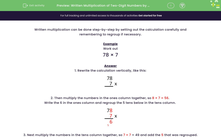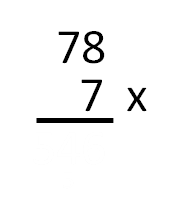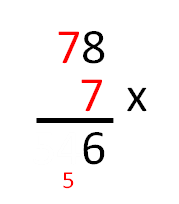# Written Multiplication of Two-Digit Numbers by One-Digit

In this worksheet, students must carry out formal written multiplications of two-digit numbers by single-digit numbers. Carrying is involved.Key stage:  KS 2

Curriculum topic:   Number: Multiplication and Division

Curriculum subtopic:   Use Written Form for Multiplication

Difficulty level:#### Worksheet Overview

Written multiplication can be done step-by-step by setting out the calculation carefully and remembering to regroup if necessary.

Example

Work out

78 × 7

1. Rewrite the calculation vertically, like this:2. Then multiply the numbers in the ones column together, so 8 × 7 = 56

Write the 6 in the ones column and regroup the 5 tens below in the tens column.3. Next multiply the numbers in the tens column together, so 7 × 7 = 49 and add the 5 that was regrouped.This gives us 49 + 5 = 54, so we write the 4 in the tens column and the 5 in the hundreds column.So      78 × 7 = 546

### What is EdPlace?

We're your National Curriculum aligned online education content provider helping each child succeed in English, maths and science from year 1 to GCSE. With an EdPlace account you’ll be able to track and measure progress, helping each child achieve their best. We build confidence and attainment by personalising each child’s learning at a level that suits them.

Get started